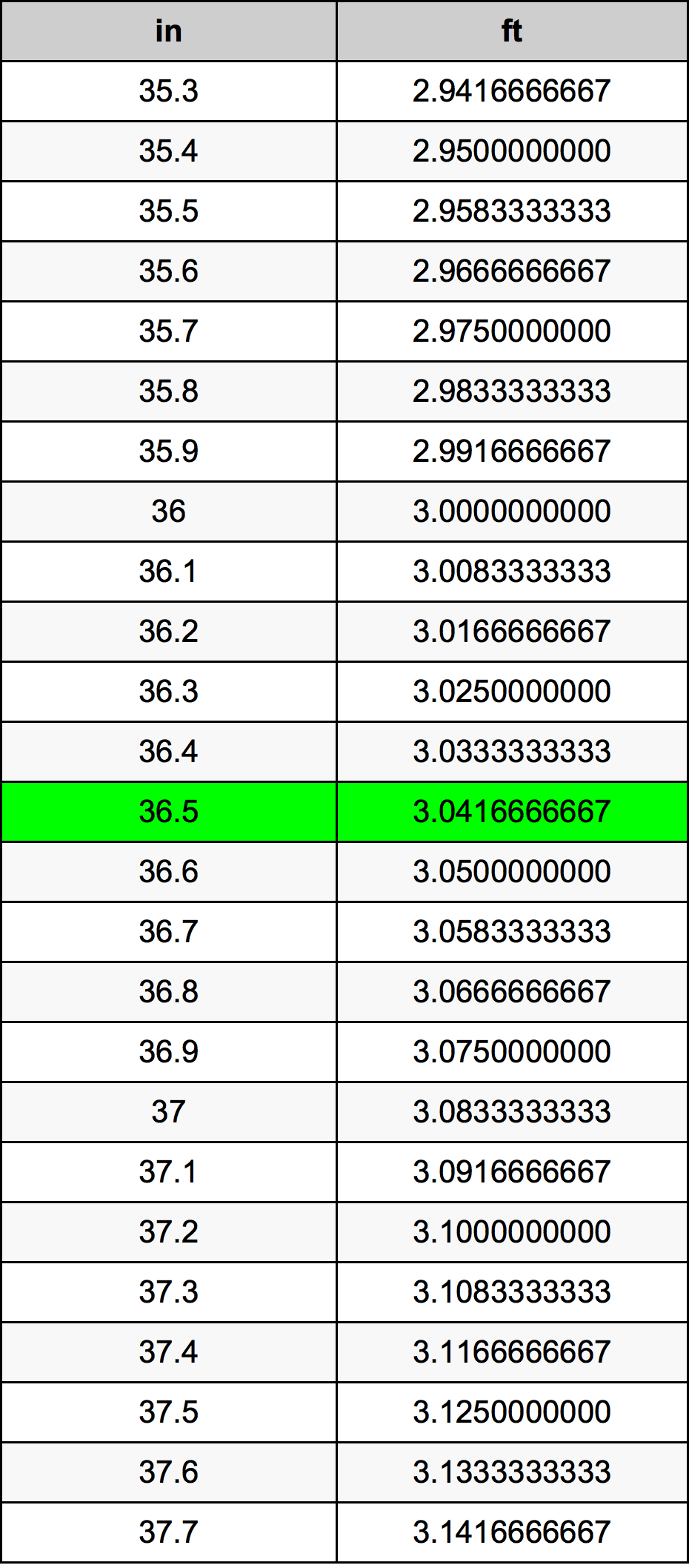Inches To Feet

# 36.5 in to ft36.5 Inches to Feet

in
=
ft

## How to convert 36.5 inches to feet?

 36.5 in * 0.0833333333 ft = 3.0416666667 ft 1 in
A common question is How many inch in 36.5 foot? And the answer is 438.0 in in 36.5 ft. Likewise the question how many foot in 36.5 inch has the answer of 3.0416666667 ft in 36.5 in.

## How much are 36.5 inches in feet?

36.5 inches equal 3.0416666667 feet (36.5in = 3.0416666667ft). Converting 36.5 in to ft is easy. Simply use our calculator above, or apply the formula to change the length 36.5 in to ft.

## Convert 36.5 in to common lengths

UnitLength
Nanometer927100000.0 nm
Micrometer927100.0 µm
Millimeter927.1 mm
Centimeter92.71 cm
Inch36.5 in
Foot3.0416666667 ft
Yard1.0138888889 yd
Meter0.9271 m
Kilometer0.0009271 km
Mile0.0005760732 mi
Nautical mile0.000500594 nmi

## What is 36.5 inches in ft?

To convert 36.5 in to ft multiply the length in inches by 0.0833333333. The 36.5 in in ft formula is [ft] = 36.5 * 0.0833333333. Thus, for 36.5 inches in foot we get 3.0416666667 ft.

## 36.5 Inch Conversion Table## Alternative spelling

36.5 Inch to Foot, 36.5 Inch in Foot, 36.5 in to Foot, 36.5 in in Foot, 36.5 Inch to Feet, 36.5 Inch in Feet, 36.5 Inches to ft, 36.5 Inches in ft, 36.5 in to ft, 36.5 in in ft, 36.5 Inches to Feet, 36.5 Inches in Feet, 36.5 Inch to ft, 36.5 Inch in ft